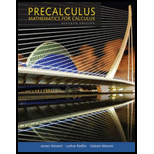Chapter 6.3, Problem 34E### Precalculus: Mathematics for Calcu...

7th Edition
James Stewart + 2 others
ISBN: 9781305071759

#### Solutions

Chapter
Section### Precalculus: Mathematics for Calcu...

7th Edition
James Stewart + 2 others
ISBN: 9781305071759
Textbook Problem

# Values of Trigonometric Functions Find the exact value of the trigonometric function.34. cos 7 π 4

To determine

To find: The value of trigonometric function.

Explanation

Given:

The given trigonometric function is cos(7π4) .

Definition:

The reference angle θ¯ is the smallest angle formed by the terminal side of θ and the x-axis.

Calculation:

The acute angle formed by the terminal side of angle 7π4 and x-axis is the reference angle θ¯ .

Figure (1)

Figure (1) shows the reference angle of 7π4 .

Since, the terminal side of given angle lies in quadrant (IV) in which cosine is positive, the reference angle is,

θ¯=2π7π4=8π7π4=π4

The value of cosine is,

cos(7π4)=cos(π4)

### Still sussing out bartleby?

Check out a sample textbook solution.

See a sample solution

#### The Solution to Your Study Problems

Bartleby provides explanations to thousands of textbook problems written by our experts, many with advanced degrees!

Get Started

## Additional Math Solutions

#### Find more solutions based on key concepts